# Hermite interpolation formula

(diff) ← Older revision | Latest revision (diff) | Newer revision → (diff)

A form of writing the polynomial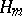of degreethat solves the problem of interpolating a functionand its derivatives at points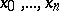, that is, satisfying the conditions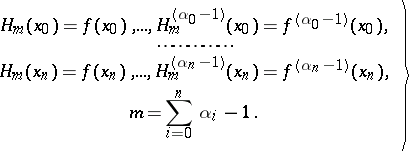(1)

The Hermite interpolation formula can be written in the form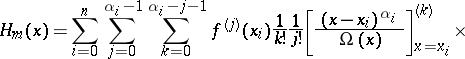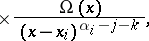where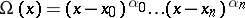.

How to Cite This Entry:
Hermite interpolation formula. Encyclopedia of Mathematics. URL: http://encyclopediaofmath.org/index.php?title=Hermite_interpolation_formula&oldid=13280
This article was adapted from an original article by M.K. Samarin (originator), which appeared in Encyclopedia of Mathematics - ISBN 1402006098. See original article